• 最优化：建模、算法与理论》、《最优化计算方法》代码展示此系列页面为配套《最优化：建模、算法与理论》、《最优化计算方法》的代码展示， 包含完整的算法和数值实验的 MATLAB 实现以及详细的注释。 有兴趣的读者...

《最优化：建模、算法与理论》、《最优化计算方法》代码展示

此系列页面为配套《最优化：建模、算法与理论》、《最优化计算方法》的代码展示， 包含完整的算法和数值实验的 MATLAB 实现以及详细的注释。 有兴趣的读者可以通过阅读代码细节理解算法及其数值表现，并且掌握其中的代码技巧。

按照书中算法与应用实例出现的顺序，将页面整理如下。其中标注“实例”的表示数值实验， 其它则为算法实现。同时，在数值实验和相应的算法的页面中都提供了相互的链接以供参考。 另外，我们还将 LASSO 问题求解与逻辑回归两个实例整理在最后。

目录

程序下载

所有算法的源文件以及其它数据文件， 请下载该压缩文件。

本系列页面所含代码适用于教学目的。在科研、生产等环境中， 读者还需选择专业的算法程序完成所需任务。

无约束优化算法

约束优化算法

复合优化算法

应用：LASSO 问题求解

LASSO 问题是在多个算法中出现的应用，现将其整理如下：

可以看出 LASSO 问题的连续化策略在其求解的过程中扮演着重要的作用。

应用：逻辑回归问题求解

参考与版权信息

刘浩洋，户将，李勇锋，文再文，最优化：建模、算法与理论(详细版)

刘浩洋，户将，李勇锋，文再文，最优化计算方法(简明版)

限于作者的知识水平，程序中难免有不妥和错误之处，恳请读者不吝批评和指正。

代码作者：

代码整理与网页制作：

展开全文数据科学和机器学习中的优化理论与算法（上）

本文希望从基础知识的角度，尽可能全地对最简单的优化理论和算法做一个小结。内容涵盖以下几个方面：优化简介、无约束优化、线搜索方法、信赖域方法、共轭梯度方法、拟牛顿方法、最小二乘问题、非线性方程、约束优化理论、非线性约束优化算法、二次规划、罚方法…

通过本文档的学习，相信你会掌握数据科学和机器学习中用到的优化基础知识，以后再遇到优化问题，就不会再困惑了。

建议收藏，方便时时查阅。

简介

数学背景

所谓的优化，在数学上说呢，就是最小化或者最大化一个函数，使得这个函数的变量满足一些约束。我们一般用 x x 向量来表示变量，称为未知数或者叫参数。 f f 叫做目标函数，它是我们想要最大化或者最小化的一个标量函数。 c i c_i 我们称之为约束函数，用来定义和 x x 必须要满足的确定的等式或者不等式约束。
优化问题标准的数学方程一般写成下述形式：

min ⁡ x ∈ R n f ( x )  subject to  c i ( x ) = 0 , i ∈ E = { 1 , … , m e } c i ( x ) ≥ 0 , i ∈ I = { m e + 1 , … , m } (1) \begin{array}{ll} {\min _{x \in \mathbb{R}^{n}}} & {f(x)} \\ {\text { subject to }} & {c_{i}(x)=0, \quad i \in \mathcal{E}=\left\{1, \ldots, m_{e}\right\}}\\ & {c_{i}(x) \geq 0, \quad i \in \mathcal{I}=\left\{m_{e}+1, \ldots, m\right\}} \end{array} \tag{1}

这里的 E \mathcal{E} 表示等式约束的指标集， I \mathcal{I} 表示不等式约束的指标集。规划问题又可分为线性规划和非线性规划，一般来说，只要目标函数或者约束函数当中有一个是非线性的，我们就称之为非线性规划。

分类

按照目标函数和约束函数的性质、变量的规模、函数的光滑性，我们可以粗略地将优化问题按如下分类：

• 连续优化和离散优化

• 全局最优化和局部最优化

• 光滑优化和非光滑优化

• 随机优化和确定性优化

• 约束优化和无约束优化：等式约束和不等式约束的指标集都为空集称为约束优化，否则称为无约束优化

• 凸优化和非凸优化

当然，还有一些别的分类方式，比如线性和非线性、可微和不可微、大规模和小规模等等。

凸性

• 一个集合 S ∈ ℜ n S \in \Re^{n} 被称为凸集，如果对于任意的两个点， x ∈ S , y ∈ S x \in S,y\in S ，对任意的 α ∈ [ 0 , 1 ] \alpha \in[0,1] ，我们有 α x + ( 1 − α ) y ∈ S \alpha x+(1-\alpha) y \in S

• 我们说 f f 是个凸函数，当它的定义域是个凸集，并且下式满足。当下式对于 x ≠ y x\neq y ，且 α \alpha 在开区间 ( 0 , 1 ) (0,1) 上严格成立（取不到等号）时，我们称 f f 是严格凸的。若 − f -f 是凸函数，那么 f f 就是一个凹函数。

f ( α x + ( 1 − α ) y ) ≤ α f ( x ) + ( 1 − α ) f ( y ) ∀ α ∈ [ 0 , 1 ] f(\alpha x+(1-\alpha) y) \leq \alpha f(x)+(1-\alpha) f(y) \quad \forall \alpha \in[0,1]

• 所谓的凸优化，一般说的是在约束优化问题中，目标函数是凸的，等式约束是线性的，且不等式约束函数是凹函数。为什么不等式约束是凹函数呢？因为我们这里定义的不等式是 c i ( x ) ≥ 0 c_{i}(x) \geq 0 ，若改成 ≤ \leq ，则是凸函数。

优化算法

优化算法一般都是一些迭代型的算法。所谓的迭代，就是先给定一个初值，然后通过一定的算法按照 x k → x k + 1 , k = 1 , 2 , … x_{k} \rightarrow x_{k+1}, \quad k=1,2, \ldots 的方式进行迭代更新。优化算法往往有一些评价指标。

• 鲁棒性（Robustness）：对于不同问题和初值都要是表现良好。

• 有效性（Efficiency）：不需要额外的时间和内存。

• 准确性（Accuracy）：能够准确地求解，不能对数据误差或者算法在数值计算时产生的舍入误差过度敏感。

除了以上几点，个人认为应该还添加上以下几点：收敛性、最优性、可扩展性、可靠性、可用性。

背景材料

向量和矩阵

• 向量的表示用 x ∈ R n : x = ( x 1 , … , x n ) T x \in \mathbb{R}^{n}: x=\left(x_{1}, \ldots, x_{n}\right)^{T}

• 向量內积：给定 x , y ∈ R n , x T y = ∑ i = 1 n x i y i x, y \in \mathbb{R}^{n}, x^{T} y=\sum_{i=1}^{n} x_{i} y_{i}

• 矩阵的表示用 A ∈ R m × n A \in \mathbb{R}^{m \times n}

• 半正定矩阵： x T A x ≥ 0 x^{T} A x \geq 0 对于任意的 x ∈ R n x \in \mathbb{R}^{n}

• 正交矩阵： Q T Q = Q Q T = I Q^{T} Q=Q Q^{T}=I

• 特征值和特征向量： A x = λ x A x=\lambda x

向量范数

• x ∈ R n  .  {x \in \mathbb{R}^{n} \text { . }}

l 1  -norm:  ∥ x ∥ 1 = ∑ i = 1 n ∣ x i ∣ l 2  -norm:  ∥ x ∥ 2 = ( ∑ i = 1 n x i 2 ) 1 / 2 = ( x T x ) 1 / 2 l ∞  -norm:  ∥ x ∥ ∞ = max ⁡ i = 1 , … , n ∣ x i ∣ \begin{array}{l} {\qquad \begin{aligned} l_{1} \text { -norm: } &\|x\|_{1}=\sum_{i=1}^{n}\left|x_{i}\right| \\ l_{2} \text { -norm: } &\|x\|_{2}=\left(\sum_{i=1}^{n} x_{i}^{2}\right)^{1 / 2}=\left(x^{T} x\right)^{1 / 2} \\ l_{\infty} \text { -norm: } &\|x\|_{\infty}=\max _{i=1, \ldots, n}\left|x_{i}\right| \end{aligned}} \end{array}

• ∥ x ∥ ∞ ≤ ∥ x ∥ 2 ≤ n ∥ x ∥ ∞ \|x\|_{\infty} \leq\|x\|_{2} \leq \sqrt{n}\|x\|_{\infty} ∥ x ∥ ∞ ≤ n ∥ x ∥ ∞ \|x\|_{\infty} \leq n\|x\|_{\infty}

• 柯西-斯瓦茨不等式： ∣ x T y ∣ ≤ ∥ x ∥ 2 ∥ y ∥ 2 \left|x^{T} y\right| \leq\|x\|_{2}\|y\|_{2}

对偶范数

∥ ⋅ ∥ \|\cdot\| 的对偶范数：

∥ x ∥ D = max ⁡ ∥ y ∥ = 1 x T y = max ⁡ y ≠ 0 x T y ∥ y ∥ \|x\|_{D}=\max _{\|y\|=1} x^{T} y=\max _{y \neq 0} \frac{x^{T} y}{\|y\|}

根据对偶范数的定义，容易知道 ∣ x T y ∣ ≤ ∥ y ∥ ∥ x ∥ D \left|x^{T} y\right| \leq\|y\|\|x\|_{D}

矩阵范数

• 给定 A ∈ R m × n , A \in \mathbb{R}^{m \times n}, 定义 ∥ A ∥ = sup ⁡ x ≠ 0 ∥ A x ∥ ∥ x ∥ \|A\|=\sup _{x \neq 0} \frac{\|A x\|}{\|x\|} ，有

∥ A ∥ 1 = max ⁡ j = 1 , … , n ∑ i = 1 m ∣ A i j ∣ ∥ A ∥ 2 = largest ⁡  eigenvalue of  ( A T A ) 1 / 2 ∥ A ∥ ∞ = max ⁡ i = 1 , … , m ∑ j = 1 n ∣ A i j ∣ \begin{array}{l} {\|A\|_{1}=\max _{j=1, \ldots, n} \sum_{i=1}^{m}\left|A_{i j}\right|} \\ {\|A\|_{2}=\operatorname{largest} \text { eigenvalue of }\left(A^{T} A\right)^{1 / 2}} \\ {\|A\|_{\infty}=\max _{i=1, \ldots, m} \sum_{j=1}^{n}\left|A_{i j}\right|} \end{array}

• Frobenius范数：

∥ A ∥ F = ( ∑ i = 1 m ∑ j = 1 n A i j 2 ) 1 / 2 \|A\|_{F}=\left(\sum_{i=1}^{m} \sum_{j=1}^{n} A_{i j}^{2}\right)^{1 / 2}

• 条件数： κ ( A ) = ∥ A ∥ ∥ A − 1 ∥ \kappa(A)=\|A\|\left\|A^{-1}\right\|

子空间

所谓子空间，给定 S ⊂ R n \mathcal{S} \subset \mathbb{R}^{n} ，若 x , y ∈ S x, y \in \mathcal{S} ，那么 α x + β y ∈ S , \alpha x+\beta y \in \mathcal{S}, 对于所有的 α , β ∈ R \alpha, \beta \in \mathbb{R}
零空间： Null ⁡ ( A ) = { w ∣ A w = 0 } \operatorname{Null}(A)=\{w | A w=0\} ，值空间： Range ⁡ ( A ) = { w ∣ w = A v  for some vector  v } \operatorname{Range}(A)=\{w | w=A v \text { for some vector } v\} ，零空间和转置的值空间的直和为全空间：Null ( A ) ⊕ (A) \oplus Range ( A T ) = R n \left(A^{T}\right)=\mathbb{R}^{n}

导数

• Frechet 可微性:
f : R n → R , f: \mathbb{R}^{n} \rightarrow \mathbb{R}, x x 处是可微的，若 ∃ g ∈ R n \exists g \in \mathbb{R}^{n} 使得：

lim ⁡ y → 0 f ( x + y ) − f ( x ) − g T y ∥ y ∥ = 0 \lim _{y \rightarrow 0} \frac{f(x+y)-f(x)-g^{T} y}{\|y\|}=0

• f f 的梯度：

g ( x ) = ∇ f ( x ) = ( ∂ f ∂ x 1 , … , ∂ f ∂ x n ) T ∈ R n g(x)=\nabla f(x)=\left(\frac{\partial f}{\partial x_{1}}, \ldots, \frac{\partial f}{\partial x_{n}}\right)^{T} \in \mathbb{R}^{n}

• f f 的 Hessian 矩阵：

H ( x ) = ∇ 2 f ( x ) = [ ∂ 2 f ∂ x i ∂ x j ] ∈ R n × n H(x)=\nabla^{2} f(x)=\left[\frac{\partial^{2} f}{\partial x_{i} \partial x_{j}}\right] \in \mathbb{R}^{n \times n}

• 链式法则 1：

d ϕ ( α ( β ) ) d β = ϕ ′ ( α ) α ′ ( β ) \frac{d \phi(\alpha(\beta))}{d \beta}=\phi^{\prime}(\alpha) \alpha^{\prime}(\beta)

• 链式法则 2： x , t ∈ R n x, t \in \mathbb{R}^{n} x = x ( t ) x=x(t) ，定义 h ( t ) = f ( x ( t ) ) h(t)=f(x(t)) ，那么

∇ h ( t ) = ∑ i = 1 n ∂ f ∂ x i ∇ x i ( t ) = ∇ x ( t ) T ∇ f ( x ( t ) ) \nabla h(t)=\sum_{i=1}^{n} \frac{\partial f}{\partial x_{i}} \nabla x_{i}(t)=\nabla x(t)^T \nabla f(x(t))

• 方向导数： D ( f ( x ) : p ) = lim ⁡ ϵ → 0 f ( x + ϵ p ) − f ( x ) ϵ = ∇ f ( x ) T p D(f(x): p)=\lim _{\epsilon \rightarrow 0} \frac{f(x+\epsilon p)-f(x)}{\epsilon}=\nabla f(x)^{T} p

收敛率

{ x k } \left\{x_{k}\right\} R n \mathbb{R}^{n} 的一个序列，收敛到 x ∗ x^{*}

• Q-线性收敛说的是存在一个常数 r ∈ ( 0 , 1 ) r\in(0,1) ，使得当 k k 充分大时，有：

∥ x k + 1 − x ∗ ∥ ∥ x k − x ∗ ∥ ≤ r \frac{\left\|x_{k+1}-x_{*}\right\|}{\left\|x_{k}-x^{*}\right\|} \leq r

• Q-超线性收敛指的是：

lim ⁡ k → ∞ ∥ x k + 1 − x ∗ ∥ ∥ x k − x ∗ ∥ = 0 \lim _{k \rightarrow \infty} \frac{\left\|x_{k+1}-x^{*}\right\|}{\left\|x_{k}-x^{*}\right\|}=0

• Q-二次收敛指的是，存在一个常数 M M ，使得当 k k 充分大时，

∥ x k + 1 − x ∗ ∥ ∥ x k − x ∗ ∥ 2 ≤ M \frac{\left\|x_{k+1}-x_{*}\right\|}{\left\|x_{k}-x^{*}\right\|^{2}} \leq M

无约束优化

f : ℜ n → ℜ f: \Re^{n} \rightarrow \Re 是一个光滑函数，无约束优化指的就是没有约束的优化。

min ⁡ x ∈ R n f ( x ) \min _{x \in \mathbb{R}^{n}} f(x)

不同说法的解

全局极小值指的是

f ( x ∗ ) ≤ f ( x )  for all  x ∈ R n f\left(x^{*}\right) \leq f(x) \quad \text { for all } x \in \mathbb{R}^{n}

局部极小值指的是存在 x ∗ x^{*} 的一个领域 N \mathcal{N}

f ( x ∗ ) ≤ f ( x )  for all  x ∈ N f\left(x^{*}\right) \leq f(x) \quad \text { for all } x \in \mathcal{N}

严格的局部极小值，

f ( x ∗ ) < f ( x )  for all  x ∈ N  with  x ≠ x ∗ f\left(x^{*}\right)<f(x) \quad \text { for all } x \in \mathcal{N} \text { with } x \neq x^{*}

孤立的局部极小值，当 x ∗ x^{*} 是领域 N \mathcal{N} 中唯一的局部极小值。

识别局部极小值

泰勒定理

首先介绍一个泰勒定理。假定 f : ℜ n → ℜ f: \Re^{n} \rightarrow \Re 是一个连续可微函数，并且 p ∈ ℜ n p \in \Re^{n} ，那么，我们有

f ( x + p ) = f ( x ) + ∇ f ( x + t p ) T p f(x+p)=f(x)+\nabla f(x+t p)^{T} p

对某个 t ∈ ( 0 , 1 ) t \in(0,1) 。进一步，如果 f f 是二次可微函数，我们有

∇ f ( x + p ) = ∇ f ( x ) + ∫ 0 1 ∇ 2 f ( x + t p ) p d t \nabla f(x+p)=\nabla f(x)+\int_{0}^{1} \nabla^{2} f(x+t p) p d t

并且

f ( x + p ) = f ( x ) + ∇ f ( x ) T p + 1 2 p T ∇ 2 f ( x + t p ) p f(x+p)=f(x)+\nabla f(x)^{T} p+\frac{1}{2} p^{T} \nabla^{2} f(x+t p) p

对某个 t ∈ ( 0 , 1 ) t \in(0,1)

一、二阶必要性条件

一阶必要性条件：如果 x ∗ x^{*} 是一个局部极小值，并且 f f x ∗ x^{*} 的某个开领域内时连续可微的，那么 ∇ f ( x ∗ ) = 0 \nabla f\left(x^{*}\right)=0
二阶必要性条件：如果 x ∗ x^{*} 是个局部极小值点，并且 f f ∇ 2 f ( x ) \nabla^{2} f(x) 存在且在 x ∗ x^{*} 的某个开领域中是连续的，那么 ∇ f ( x ∗ ) = 0 \nabla f\left(x^{*}\right)=0 并且 ∇ 2 f ( x ∗ ) \nabla^{2} f\left(x^{*}\right) 是个半正定的矩阵。
稳定点表示满足 ∇ f ( x ∗ ) = 0 \nabla f\left(x^{*}\right)=0 x ∗ x^{*} 点。

二阶充分性条件

假定 ∇ 2 f ( x ) \nabla^{2} f(x) x ∗ x^{*} 的开领域中是连续的， ∇ f ( x ∗ ) = 0 \nabla f\left(x^{*}\right)=0 ∇ 2 f ( x ∗ ) \nabla^{2} f\left(x^{*}\right) 正定的，那么 x ∗ x^{*} f f 的一个严格极小值点。

从这个条件中可以看出，这里必要性条件，有个更高的要求，要求二阶导是正定的，得到的结论也更强，是严格的局部极小值。这个条件也只是充分的，而不是必要的条件，也就是说存在严格局部极小值点，不一定满足正定这个条件，但是半正定一定要满足，这是必要性条件告诉我们的。

如果 f f 是个凸函数，那么局部极小值就是全局极小值。进一步，如果 f f 是可微的，那么任何的稳定点都是全局极小值点。对于迭代方法，我们一般找到的是梯度消失的点，也就是稳定点。

无约束优化问题的常用算法

求解这种无约束优化问题，常用的有线搜索方法和信赖域方法，这两种方法后面会详细地提到。简单地提一句，线搜索方法其实就是每次迭代都沿着一个方向（类似于一根线）走一定的步长，等走到那个点了，再用类似的方法走到下一个点。

信赖域方法，我国的第一代留学生------孙悟空，就曾经用过此法。唐僧取经那一会儿，总归要有人出去化缘，八戒这货喜欢偷懒，经常赖着不愿意出去要饭，师傅总是要吃饭哪，所以，只能猴哥出去化缘。猴子出去了，但是又怕师傅会被妖怪吃掉，只能画个圈圈，把师徒三人圈在圈圈里面，并叮嘱他们不要出来，免得要妖怪吃掉。这个圈圈啊，其实就是我们所说的"信赖域"。孙悟空的法力值就那么大，圈圈不能画太大，画太大就没什么威力了，容易被妖怪打破。师徒三人呢，活动也不能超过这个范围，如果跑出这个范围呢，就会被妖怪吃掉。如果被妖怪吃掉了，就取不成经，取不成经，就不"收敛"了

线搜索方法简述

所谓的线搜索方法，就是说在每一次迭代我们得先找一个搜索方向 p k p_k ，然后沿着搜索方向走一步，

x k + 1 = x k + α k p k x_{k+1}=x_{k}+\alpha_{k} p_{k}

这里的 α k \alpha_k 我们一般称之为步长，在机器学习当中，我们一般也叫它学习率。算法描述如图。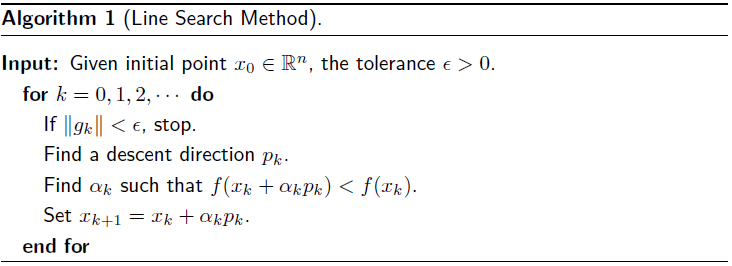梯度下降方向

如果选择下降方向呢，一个比较流行的选择就是最速下降方向，也就是负梯度方向。 − ∇ f k -\nabla f_{k} 是当前点 f f 下降最快的方向，需要注意这只是当前点的下降最快的方向，所以它不一定是收敛最快的方向。为什么负梯度方向是下降最快的方向呢？从泰勒展开就可以看出来了。

f ( x k + α p ) = f ( x k ) + α p T ∇ f k + α 2 2 p T ∇ 2 f ( x k + t p ) p f\left(x_{k}+\alpha p\right)=f\left(x_{k}\right)+\alpha p^{T} \nabla f_{k}+\frac{\alpha^{2}}{2} p^{T} \nabla^{2} f\left(x_{k}+t p\right) p

忽略二次项的高阶小量，欲令前后两步的差值尽量大， p p 自然取为负梯度方向。一般满足 p T ∇ f k < 0 p^{T} \nabla f_{k}<0 的方向，我们都叫下降方向。

在机器学习中，我们经常提到随机梯度下降。我们知道，在做有监督的问题中，有一个损失函数，用来衡量模型的好坏。在回归问题中，如果我们已经知道方程的形式，我们可以做出问题的损失函数。那么我们要做的就是寻找方程的最佳参数，即寻找参数，使得损失函数函数达到最小。乍一听，这就是个优化问题。当然可以对参数的各个分量求导，最后解方程组。问题是，你能确定最后的方程组好解？一般的数值解法有梯度下降法、牛顿法、拟牛顿方法以及信赖域方法等等。

梯度下降方法简单易行（大部分情况下还是收敛挺快），可是当数据量比较大时，梯度下降的计算就特别大。为解决大数据量的优化求解，我们一般可以采用随机梯度下降。
所谓的随机梯度下降，就是相比梯度下将，我们不选择全部的数据点，而是每次随机选择一个或者若干个数据点来构建损失函数，接着再用梯度下降方法求解。看起来很不靠谱的样子，事实上多做几次这个操作，它是可以收敛的最优解的某个邻域的。

牛顿和拟牛顿方向

做二阶泰勒近似，

f ( x k + p ) ≈ f k + p T ∇ f k + 1 2 p T ∇ 2 f k p ≡ m k ( p ) f\left(x_{k}+p\right) \approx f_{k}+p^{T} \nabla f_{k}+\frac{1}{2} p^{T} \nabla^{2} f_{k} p \equiv m_{k}(p)

我们希望找到一个 p p ，使得 m k m_k 最小，求解这个二次函数的最小值，可得，

p k N = − ( ∇ 2 f k ) − 1 ∇ f k p_{k}^{N}=-\left(\nabla^{2} f_{k}\right)^{-1} \nabla f_{k}

这便是传说中的牛顿方向。使用牛顿方向，就称为牛顿方法。

有时候，二阶 Hessian 阵不是那么好算，我们会找一个 B k B_k 去逼近 ∇ 2 f k \nabla^{2} f_{k} ，就得到了拟牛顿方向，

p k = − B k − 1 ∇ f k p_{k}=-B_{k}^{-1} \nabla f_{k}

那么这个近似方向 B k B_k 怎么选呢？不要着急，后面还有专门的章节来讨论拟牛顿方法。比如说，BFGS方法， B k B_k 就是这样迭代的，

B k + 1 = B k − B k s k s k T B k s k T B k s k + y k y k T y k T s k B_{k+1}=B_{k}-\frac{B_{k} s_{k} s_{k}^{T} B_{k}}{s_{k}^{T} B_{k} s_{k}}+\frac{y_{k} y_{k}^{T}}{y_{k}^{T} s_{k}}

这里， s k = x k + 1 − x k , y k = ∇ f k + 1 − ∇ f k s_{k}=x_{k+1}-x_{k}, \quad y_{k}=\nabla f_{k+1}-\nabla f_{k} ，不知所以然，没关系，后面还会慢慢提这个事情。简单地说， B k B_k 在满足割线方程 B k + 1 s k = y k B_{k+1} s_{k}=y_{k} 的前提下，再满足一些别的约束，就能导出不同的关于 B k B_k 的迭代公式。

非线性共轭梯度方向

当然，除了上述提到的最速下降方向、牛顿和拟牛顿方向，还有一些其他的线搜索方向。非线性共轭梯度方法诱导的方向就是其中之一。

p k = − ∇ f ( x k ) + β k p k − 1 p_{k}=-\nabla f\left(x_{k}\right)+\beta_{k} p_{k-1}

这是负梯度方向和前一个搜索方向做的一个组合，这里的 β k \beta_k 是保证 p k p_k p k − 1 p_{k-1} 共轭的一个常数。什么叫共轭，不急，后面还会详细说。

步长选择

不管是负梯度方向，还是牛顿方向，我们只是选择了方向。步长如何选取呢？一个理想的选择，就是沿着线搜索方向，找到函数最小的点对应的步长作为搜索步长。即最小化如下函数：

ϕ ( α ) = f ( x k + α p k ) , α > 0 \phi(\alpha)=f\left(x_{k}+\alpha p_{k}\right), \quad \alpha>0

因为终归是在这个方向上找到了精确的最小值点，所以一般就称为精确线搜索步长。对应于精确线搜索的，就是非精确线搜索。也就是找一堆步长值去试，直到满足一定的终止条件。
使用非精确线搜索步长，步长一定要满足一定的条件。一个简单的例子就是 f = x 2 f=x^2 ，如果步长没有选取好，即使是梯度下降方法，迭代也有可能在最小值附近一左一右跑，呈"之"字型荡来荡去，收敛极慢，或者索性就不收敛了。就不画图了，应该能想象的到。为了避免这种情况，我们需要对步长施加一些充分下降条件。充分下降条件就是保证选定的步长，使得 f f 值是下降的，

f ( x k + α p k ) ≤ f ( x k ) + c 1 α ∇ f k T p k : = l ( α ) f\left(x_{k}+\alpha p_{k}\right) \leq f\left(x_{k}\right)+c_{1} \alpha \nabla f_{k}^{T} p_{k}:=l(\alpha)

这里的 c 1 ∈ ( 0 , 1 ) c_1 \in (0,1) ，一般可以取 1 0 − 4 10^{-4} 。这个条件告诉我们， f f 的下降要和步长和方向导数成比例。满足充分下降条件还是不够的，因为只要足够小的步长 α \alpha 都满足这个条件，我们还需要一些条件筛掉不合理的小步长。于是便有了曲率条件，

∇ f ( x k + α k p k ) T p k ≥ c 2 ∇ f k T p k \nabla f\left(x_{k}+\alpha_{k} p_{k}\right)^{T} p_{k} \geq c_{2} \nabla f_{k}^{T} p_{k}

这里 c 2 ∈ ( c 1 , 1 ) c_{2} \in\left(c_{1}, 1\right) ，在牛顿和拟牛顿方法中，一般取为0.9，非线性CG方法中，一般取为0.1。 c 1 c_1 来自于充分下降条件。如果定义 ϕ ( α ) = f ( x k + α p k ) \phi(\alpha)=f\left(x_{k}+\alpha p_{k}\right) ，那么曲率条件也可以写成 ϕ ′ ( α k ) ≥ c 2 ϕ ′ ( 0 ) \phi^{\prime}\left(\alpha_{k}\right) \geq c_{2} \phi^{\prime}(0) 。也就是说走一步之后，原下降方向就要离负梯度方向更远一些，下降得就没那么厉害一些。

所谓的 Wolfe 条件就是把充分下降条件和曲率条件放在一块，

f ( x k + α k p k ) ≤ f ( x k ) + c 1 α k ∇ f k T p k ∇ f ( x k + α k p k ) T p k ≥ c 2 ∇ f k T p k \begin{aligned} f\left(x_{k}+\alpha_{k} p_{k}\right) & \leq f\left(x_{k}\right)+c_{1} \alpha_{k} \nabla f_{k}^{T} p_{k} \\ \nabla f\left(x_{k}+\alpha_{k} p_{k}\right)^{T} p_{k} & \geq c_{2} \nabla f_{k}^{T} p_{k} \end{aligned}

强 Wolfe 条件，就是在曲率条件上加个绝对值，它要求 α k \alpha_k 满足，

f ( x k + α k p k ) ≤ f ( x k ) + c 1 α k ∇ f k T p k ∣ ∇ f ( x k + α k p k ) T p k ∣ ≤ c 2 ∣ ∇ f k T p k ∣ \begin{aligned} f\left(x_{k}+\alpha_{k} p_{k}\right) & \leq f\left(x_{k}\right)+c_{1} \alpha_{k} \nabla f_{k}^{T} p_{k} \\ \left|\nabla f\left(x_{k}+\alpha_{k} p_{k}\right)^{T} p_{k}\right| & \leq c_{2}\left|\nabla f_{k}^{T} p_{k}\right| \end{aligned}

同样， 0 < c 1 < c 2 < 1 0<c_{1}<c_{2}<1 。说了 Wolfe 条件和强 Wolfe 条件，那么是否一定能找到这种苛刻条件的步长呢？答案是肯定的。定理表明，对于光滑有界的函数而言，总是能找到满足 Wolfe 条件和强 Wolfe 条件的步长的。

还有一个条件叫做 Goldstein 条件，它也能保证充分下降，且步长不太小。

f ( x k ) + ( 1 − c ) α k ∇ f k T p k ≤ f ( x k + α k p k ) ≤ f ( x k ) + c α k ∇ f k T p k f\left(x_{k}\right)+(1-c) \alpha_{k} \nabla f_{k}^{T} p_{k} \leq f\left(x_{k}+\alpha_{k} p_{k}\right) \leq f\left(x_{k}\right)+c \alpha_{k} \nabla f_{k}^{T} p_{k}

这里 0 < c < 1 2 0<c<\frac{1}{2} ，右半部分是充分下降条件，左半部分保证了步长不太小。

我们可以利用充分下降条件，构造回溯算法。所谓的回溯法，就是开始的时候，选个比较大的步长，慢慢减小之，直到它满足了充分下降条件，立即停止，这样，也使得步长不太小了。

线搜索方法的收敛性

介绍一下 Zoutendijk 条件： ∑ k ≥ 0 cos ⁡ 2 θ k ∥ ∇ f k ∥ 2 < ∞ \sum_{k \geq 0} \cos ^{2} \theta_{k}\left\|\nabla f_{k}\right\|^{2}<\infty 。这里的 θ k \theta_k 是最速下降方向和线搜索方向的夹角，即

cos ⁡ θ k = − ∇ f k T p k ∥ ∇ f k ∥ ∥ p k ∥ \cos \theta_{k}=\frac{-\nabla f_{k}^{T} p_{k}}{\left\|\nabla f_{k}\right\|\left\|p_{k}\right\|}

有定理表明，在一些本质条件下，若 Wolfe 条件被满足，那么 Zoutendijk 条件总是成立的。Zoutendijk 条件表明 cos ⁡ 2 θ k ∥ ∇ f k ∥ 2 → 0 \cos ^{2} \theta_{k}\left\|\nabla f_{k}\right\|^{2} \rightarrow 0 。容易知道，不管是梯度下降方法还是牛顿方法（ B k B_k 正定）， cos ⁡ θ k \cos \theta_{k} 都是有下界的，也就是梯度方向和搜索方向总是不垂直，那么必然有，

lim ⁡ k → ∞ ∥ ∇ f k ∥ = 0 \lim _{k \rightarrow \infty}\left\|\nabla f_{k}\right\|=0

即算法至少收敛到一个稳定点，我们说收敛到梯度为0的点，叫做"全局收敛"的。下面给出改进的线搜索牛顿法的一个算法描述，如图。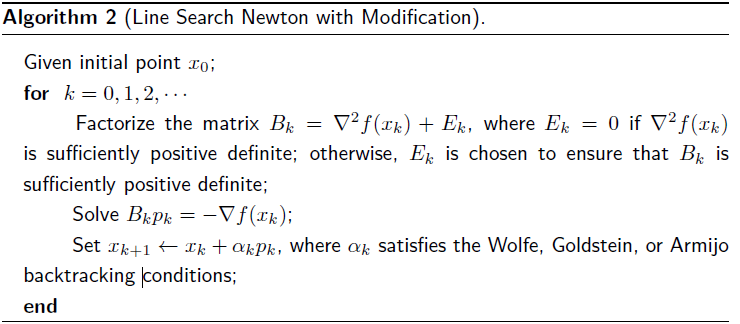这里保证 B k B_k 正定，是为了保证收敛。事实上，定理表明，只要保证 B k B_k 的条件数足够小就可以了。

最速下降方法的收敛率依赖于 f f 的 Hessian 的条件数，一般来说不超过线性收敛的速度，一些特殊情况下，收敛速度慢得可怜。对于牛顿方法，满足一定的不太严苛的条件，一般是全局收敛的。若满足二阶充分性条件，当初值选得足够靠近真值时，一般就是二次收敛的。同样满足二阶充分性条件，拟牛顿方法就会差一些，它是超线性收敛的。

信赖域方法

信赖域方法（Trust-region methods）又称为TR方法，它是一种最优化方法，能够保证最优化方法总体收敛。现今，信赖域算法广泛应用于应用数学、物理、化学、工程学、计算机科学、生物学与医学等学科。相信在不远将来，信赖域方法会在更广泛多样的领域有着更深远的的发展。这里简单以介绍信赖域狗腿（dogleg）方法，让大家体会一下什么叫信赖域方法，希望能为其他专业诸如机器学习的同学在使用优化工具时提供一个参考。如前所述，信赖域方法其实并不新鲜，我国的第一代留学生------孙悟空，早就用过此法，也就是我们常说的"画个圈圈诅咒你"。

原理描述

考虑模型问题：

min ⁡ p ∈ R n m ( p ) = f + g T p + 1 2 p T B p ,  s.t.  ∥ p ∥ ≤ Δ \min _{p \in \mathbb{R}^{n}} m(p)=f+g^{T} p+\frac{1}{2} p^{T} B p, \quad \text { s.t. }\|p\| \leq \Delta

它具有全局最优解：

p B = − B − 1 g p^{B}=-B^{-1} g

该问题沿着负梯度方向，具有全局最优解：

p U = − g T g g T B g g p^{U}=-\frac{g^{T} g}{g^{T} B g} g

由于信赖域的限制，全局最优解可能落在信赖域之外。我们依据两个全局最优点是否落于信赖域中，来决定下降方向。

• 当B点在信赖域内部时，取方向为 p U p^U 方向， x k + 1 x_{k+1} 点为B点：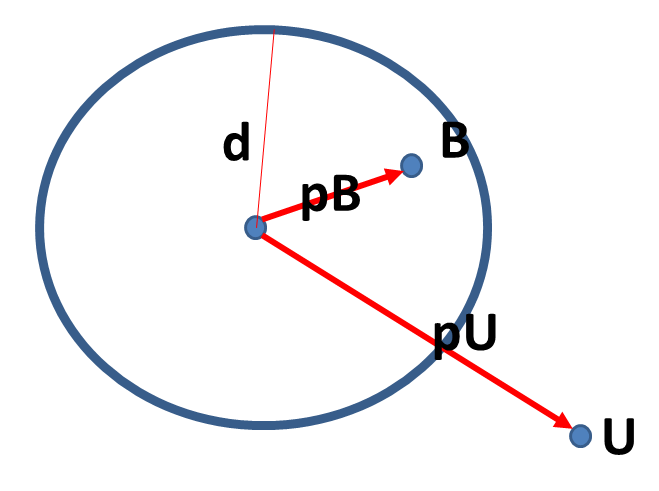• 当B点和U点都在信赖域外时，取下一个迭代点为 p U p^U 和信赖域边界的交点：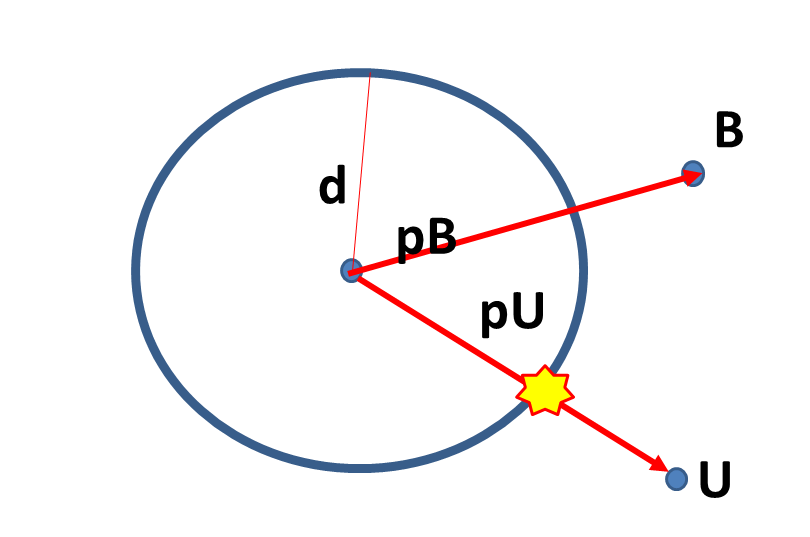• 当B点在外，U点在内时，去BU连线和信赖域边界的交点：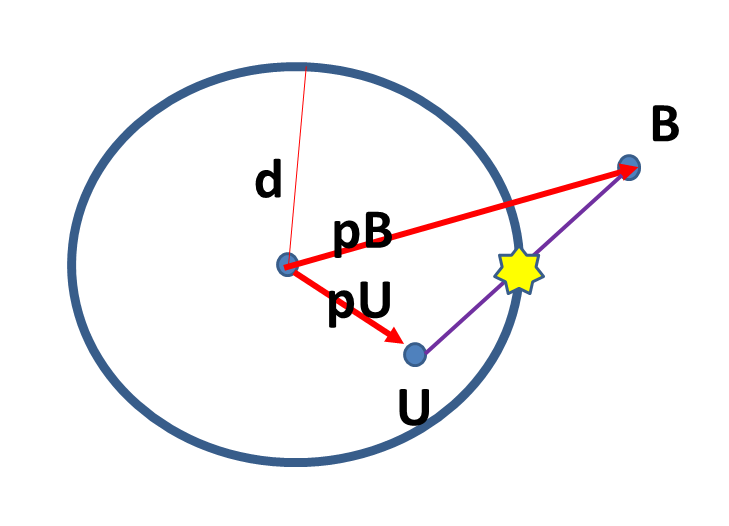我们可以用一个参数 τ \tau 将这三种情况统一为一个表达式，即下降取值为：

p ~ ( τ ) = { τ p U , 0 ≤ τ ≤ 1 p U + ( τ − 1 ) ( p B − p U ) , 1 ≤ τ ≤ 2 \tilde{p}(\tau)=\left\{\begin{array}{ll} {\tau p^{U},} & {0 \leq \tau \leq 1} \\ {p^{U}+(\tau-1)\left(p^{B}-p^{U}\right),} & {1 \leq \tau \leq 2} \end{array}\right.

这里的 τ \tau 是如何取的呢？通过简单的数学推导，我们知道它可以这样来取，刚好满足上面的三种情况。简单地说，第一种情况， τ \tau 取2，第二种情况， τ \tau 取为信赖域半径和 p U p^U 长的比值，第三种情况，要求那个交点，我们就要求一元二次方程的一个根，这可以用求根公式算得。

算法步骤

总的算法框架如图。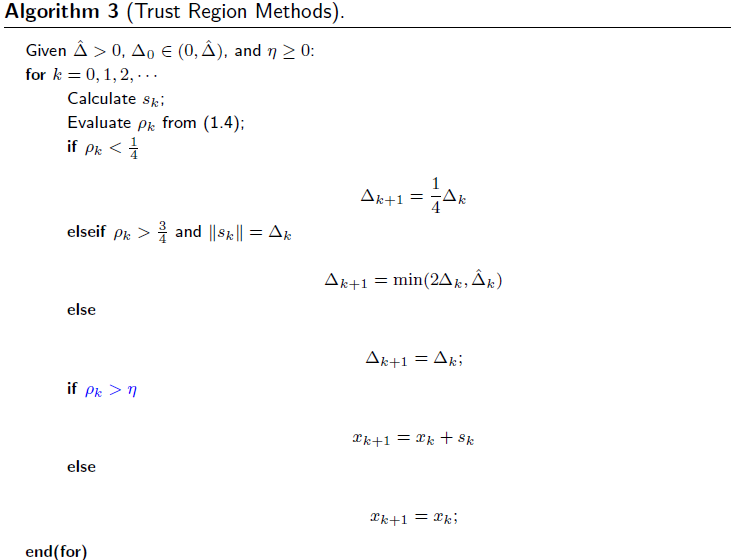τ \tau s k = x k + 1 − x k s_k = x_{k+1} - x_k 的计算就是使用上面提到的狗腿方法。下降比 ρ k \rho_k 的计算表达式为：

ρ k = f ( x k ) − f ( x k + s k ) m k ( 0 ) − m k ( s k ) \rho_{k}=\frac{f\left(x_{k}\right)-f\left(x_{k}+s_{k}\right)}{m_{k}(0)-m_{k}\left(s_{k}\right)}

所以，到这事情就很明了了。一个简单的代码如下：

function [x,k] = trust_region1(varargin)
%帮助信息：
%clc
%clear
%输入参数为options = struct('f',f,'x0',[0;0],'Delta',1,'Delta0',0.1,'eta',1/4);
if nargin < 1, help(mfilename),
f = @(x1,x2)100*(x2-x1^2)^2 + (1-x1)^2;
options = struct('f',f,'x0',[0;0],'Delta_hat',10,'Delta0',0.1,'eta',0);
fprintf('使用默认参数…… \n \n');
end

f = options.f;
syms x1 x2;
f_s = f(x1,x2);
x0 = options.x0;
Delta_hat = options.Delta_hat;
Delta0 = options.Delta0;
eta = options.eta;
df = diff_handle(f_s);
B = hessian(f_s);
Delta = Delta0;
x = x0;
step = 100;
for k = 0:step-1
x
fk = f(x(1),x(2));
dfk = df(x(1),x(2));
Bk = subs(B,{x1,x2},{x(1),x(2)});
Bk = double(Bk);
sk = cal_sk(dfk,Bk,Delta);
m = @(p) fk + dfk'*p + 1/2*p'*Bk*p;
rho_k = cal_rho_k(f,m,x,sk);
if rho_k < 1/4
Delta = 1/4*Delta;
elseif rho_k > 3/4 && abs(norm(sk,2) - Delta)<1.0e-10;
Delta = min(2*Delta,Delta_hat);
else
%不做任何操作；
end

if rho_k > eta
x = x + sk;
else
%不做任何操作；
end
end

end

function df = diff_handle(f_s)
syms x1 x2;
df = [diff(f_s,x1); diff(f_s,x2)];
df = matlabFunction(df);
end

function tau = cal_tau(pB,pU,Delta)
npB = sqrt(pB'*pB);
npU = sqrt(pU'*pU);
if npB <= Delta
tau = 2;
elseif npU >= Delta
tau = Delta/npU;
else
pB_U = pB-pU;
tau = (-pU'*pB_U+sqrt((pU'*pB_U)^2-pB_U'*pB_U*(pU'*pU-Delta^2)))/(pB_U'*pB_U);
tau = tau + 1;
end
end
% temp = conj(dfk')*Bk*dfk;
% if  temp<= 0
%     tau = 1;
% else
%     tau = min(norm(dfk,2)/temp,1);

function sk = cal_sk(dfk,Bk,Delta)
pU = -(conj(dfk')*dfk)/(conj(dfk')*Bk*dfk).*dfk;
pB = -Bk^(-1)*dfk;
tau = cal_tau(pB,pU,Delta);
if tau >=0 && tau <=1
sk = tau*pU;
elseif tau >= 1 && tau <=2
sk = pU + (tau-1)*(pB-pU);
else
error('tau的值不能为%f',tau);
end

end

function rho_k = cal_rho_k(f,m,x,sk)
rho_k = (f(x(1),x(2)) - f(x(1)+sk(1),x(2)+sk(2)))/((m([0;0])-m(sk)));
end

一个例子

使用狗腿方法来求解信赖域子问题，应用这个方法来最小化下述函数。这里的 B k = ∇ 2 f ( x k ) B_k = \nabla^2 f(x_k) 。使用信赖域方法的更新规则更新。这里给出前两步迭代结果。

f ( x ) = 100 ( x 2 − x 1 2 ) 2 + ( 1 − x 1 ) 2 f(x)=100\left(x_{2}-x_{1}^{2}\right)^{2}+\left(1-x_{1}\right)^{2}

选定的参数为：信赖域上界取为10，信赖域初始半径取为0.1， η \eta 取为0。在此参数下，迭代的前两个 x x 值为： x 0 = [ 0.1000 , 0 ] , x 1 = [ 0.2933 , 0.0513 ] x_0=[0.1000,0],x_1=[0.2933,0.0513]

在一些条件的保证下，信赖域方法能够收敛到问题的一个稳定点。如果在局部能够满足二阶充分性条件，那么信赖域方法在这个局部，能有一个超线性收敛的速度。在信赖域方法，还有一个相场被提到的概念叫"柯西点"。所谓的柯西点，我们用 s k c s_{k}^{c} 来表示，它是在信赖域约束下的一个负梯度方向最小值点，

s k c = arg ⁡ min ⁡ m k ( p )  s.t.  p = − τ ∇ f k ∥ p ∥ ≤ Δ k , τ ≥ 0 \begin{array}{rl} {s_{k}^{c}=\arg \min } & {m_{k}(p)} \\ {\text { s.t. }} & {p=-\tau \nabla f_{k}} \\ {} & {\|p\| \leq \Delta_{k}, \tau \geq 0} \end{array}

当然，信赖域方法也存在一些问题。比如说如果目标函数对某一个变量非常敏感，而对其他变量相对不敏感，也就是最优值落在一个狭长的椭圆区域里面，那么我们就说问题是 poor scaling 的，这时候，信赖域方法就会出一些问题。也有一些处理的方法，暂且不提。

展开全文大数据 人工智能 优化方法 机器学习 最小值
• 书名最优化：理论、计算应用作者薛毅出版社科学出版社出版时间2019年02月01日页数351 页定价128 元开本B5装帧平装ISBN9787030605238最优化：理论、计算应用内容简介编辑语音本书包括最优化理论、计算...

最优化：理论、计算与应用

语音

编辑

锁定

讨论

上传视频

《最优化：理论、计算与应用》是2019年科学出版社出版的图书，作者是薛毅。

书    名

最优化：理论、计算与应用

作    者

薛毅

出版社

科学出版社

出版时间

2019年02月01日页    数

351 页

定    价

128 元

开    本

B5

装    帧

平装

ISBN

9787030605238

最优化：理论、计算与应用内容简介

编辑

语音

本书包括最优化理论、计算和应用三个方面的内容，共6章，分别是最优化问题概述、一维搜索与信赖域方法、无约束最优化方法、非线性方程与最小二乘问题、线性规划、约束最优化方法。将最优化的理论、计算和应用结合在一起是本书最大的特点，其目的是让学习者掌握求解最优化问题的基本理论，理解相关算法的设计思想，了解最优化问题的求解过程，学会使用MATLAB软件(优化工具箱中的函数)计算最优化问题。

最优化：理论、计算与应用图书目录

编辑

语音

目 录

第1章 最优化问题概述 1

1.1 最优化问题的数学模型与分类 1

1.2 最优化问题 2

1.2.1 无约束最优化问题 2

1.2.2 线性规划问题 3

1.2.3 二次规划问题 4

1.2.4 约束优化问题 6

1.3 无约束问题的最优性条件 7

1.3.1 无约束问题的最优解 7

1.3.2 最优性条件 9

1.4 约束问题的最优性条件 13

1.4.1 约束问题的全局解与局部解 13

1.4.2 约束问题最优解的最优性条件 14

1.5 凸集与凸函数 21

1.5.1 凸集 22

1.5.2 凸函数 25

1.6 约束问题最优性条件的证明 28

1.6.1 一阶必要条件的证明 28

1.6.2 二阶充分条件的证明 33

1.7 MATLAB优化工具箱 35

1.7.1 MATLAB概述 35

1.7.2 MATLAB优化工具箱概述 35

1.7.3 MATLAB优化工具箱中的函数 36

习题1 37

第2章 一维搜索与信赖域方法 42

2.1 求解无约束问题的结构 42

2.1.1 一维搜索策略的下降算法 42

2.1.2 信赖域方法 42

2.2 一维搜索 43

2.2.1 精确一维搜索算法 43

2.2.2 非精确一维搜索算法 46

2.2.3 正定二次函数的一维搜索方法 48

2.3 下降算法的收敛性 49

2.3.1 算法的收敛性 49

2.3.2 算法的收敛速率 52

2.3.3 算法的二次终止性 52

2.4 信赖域方法 53

2.4.1 信赖域方法的基本结构 53

2.4.2 求解信赖域子问题的dogleg方法 54

2.4.3 信赖域方法的全局收敛性 55

2.5 自编的MATLAB程序 57

2.5.1 一维搜索程序 57

2.5.2 求解信赖域子问题折线方法 61

2.6 MATLAB优化工具箱中的函数 63

2.7 一维优化问题的应用 64

2.7.1 路灯照明问题 65

2.7.2 极大似然估计 66

习题2 67

第3章 无约束最优化方法 68

3.1 算法 68

3.1.1 最速下降法的收敛性质 68

3.1.2 算法的收敛性质 70

3.2 共轭梯度法 74

3.2.1 线性共轭梯度法 74

3.2.2 非线性共轭梯度法 79

3.2.3 共轭梯度法的收敛性质 82

3.3 Newton法 85

3.3.1 精确Newton法 85

3.3.2 Newton法的收敛性质 87

3.3.3 非精确Newton法 88

3.3.4 带有一维搜索的Newton法 89

3.3.5 信赖域Newton法 91

3.4 拟Newton法 94

3.4.1 秩1修正公式 95

3.4.2 秩1修正公式的性质 98

3.4.3 秩2修正公式 100

3.4.4 秩2修正公式的性质 105

3.4.5 信赖域算法 110

3.5 自编的MATLAB程序 111

3.5.1 最速下降法 111

3.5.2 共轭梯度法 112

3.5.3 Newton法 113

3.5.4 拟Newton法 114

3.5.5 信赖域方法 115

3.6 MATLAB优化工具箱中的函数 118

3.6.1 多变量极小化函数 118

3.6.2 设置优化参数 121

3.7 无约束最优化问题的应用 125

3.7.1 选址问题 125

3.7.2 曲线拟合问题 126

3.7.3 药物浓度的测定 126

习题3 129

4.1 求解非线性方程组 133

4.1.1 非线性方程求根 133

4.1.2 非线性方程组求解 136

4.2 超定方程组求解与最小二乘问题 143

4.2.1 线性最小二乘问题 144

4.2.2 Gauss-Newton法 146

4.2.3 Levenberg-Marquardt方法 149

4.2.4 拟Newton法 152

4.3 自编MATLAB程序 153

4.3.1 求解非线性方程的方法 153

4.3.2 求解非线性方程组的方法 156

4.3.3 求解非线性最小二乘问题的算法 161

4.4 MATLAB优化工具箱中的函数 167

4.4.1 求解非线性方程 (组) 167

4.4.2 求解线性最小二乘问题 170

4.4.3 求解非线性最小二乘问题 174

4.5 非线性方程(组)的应用 177

4.5.1 求极值问题 177

4.5.2 GPS定位问题 179

4.6 最小二乘问题的应用 182

4.6.1 曲线拟合问题 182

4.6.2 GPS定位问题(续) 182

习题4 184

第5章 线性规划 187

5.1 线性规划的数学模型 187

5.1.1 线性规划的实例 187

5.1.2 线性规划的标准形式 190

5.1.3 线性规划的图解法 191

5.2 求解线性规划问题的单纯形法 194

5.2.1 基本单纯形法 194

5.2.2 单纯形表 197

5.2.3 两阶段方法 199

5.2.4 改进单纯形法 203

5.3 线性规划的对偶问题 205

5.3.1 对偶线性规划 205

5.3.2 线性规划的对偶理论 208

5.3.3 对偶单纯形法 211

5.4 内点算法概述 215

5.4.1 算法复杂性的基本概念 215

5.4.2 单纯形法的复杂性 215

5.4.3 内点算法简介 216

5.5 自编MATLAB程序 217

5.5.1 从基本可行解开始的单纯形法 217

5.5.2 两阶段方法 218

5.5.3 从正则解开始的对偶单纯形法 220

5.6 MATLAB优化工具箱中的函数 222

5.6.1 linprog函数 222

5.6.2 Lagrange乘子的意义 224

5.6.3 intlinprog函数 227

5.7 线性规划问题的应用 230

5.7.1 城市规划 230

5.7.2 投资 233

5.7.3 生产计划与库存控制 236

5.7.4 人力规划 244

5.7.5 最小覆盖 246

5.8 用线性规划求解图论中的问题 248

5.8.1 运输问题 248

5.8.2 最优指派问题 250

5.8.3 最短路问题 253

5.8.4 最大流问题及应用 258

习题5 264

第6章 约束最优化方法 273

6.1 二次规划问题 273

6.1.1 二次规划的基本性质 273

6.1.2 等式二次规划问题 274

6.1.3 求解凸二次规划的有效集法 280

6.2 罚函数方法 286

6.2.1 二次罚函数方法 286

6.2.2 l1 模罚函数方法 294

6.2.3 乘子罚函数法 297

6.2.4 一般等式约束问题的乘子罚函数法 302

6.2.5 一般约束问题的乘子罚函数法 304

6.3 序列二次规划方法 308

6.3.1 Lagrange-Newton法 308

6.3.2 一般约束问题的序列二次规划方法 310

6.4 序列二次规划的信赖域方法 316

6.4.1 求解等式约束问题的信赖域算法 316

6.4.2 求解一般约束问题的信赖域算法 320

6.5 MATLAB优化工具箱中的函数 323

6.5.1 求解二次规划问题 323

6.5.2 求解一般约束问题 327

6.6 约束最优化问题的应用 331

6.6.1 投资组合问题 331

6.6.2 选址问题 332

习题6 334

习题参考答案 339

参考文献 347

索引 348

MATLAB函数索引 352

MATLAB自编函数索引 353

词条图册

更多图册

参考资料

1.

最优化：理论、计算与应用

．科学出版社[引用日期2020-02-20]

展开全文• matlab作业—遗传算法与优化问题.doc 遗传算法与优化问题一、问题背景实验目的遗传算法(GeneticAlgorithm—GA)，是模拟达尔文的遗传选择和自然淘汰的生物进化过程的计算模型，它是由美国Michigan大学的J.Holland...matlab作业—遗传算法与优化问题.doc

遗传算法与优化问题一、问题背景与实验目的遗传算法(GeneticAlgorithm—GA)，是模拟达尔文的遗传选择和自然淘汰的生物进化过程的计算模型，它是由美国Michigan大学的J.Holland教授于1975年首先提出的。遗传算法作为一种新的全局优化搜索算法，以其简单通用、鲁棒性强、适于并行处理及应用范围广等显著特点，奠定了它作为21世纪关键智能计算之一的地位。1．遗传算法的基本原理遗传算法的基本思想正是基于模仿生物界遗传学的遗传过程。它把问题的参数用基因代表，把问题的解用染色体代表(在计算机里用二进制码表示)，从而得到一个由具有不同染色体的个体组成的群体。这个群体在问题特定的环境里生存竞争，适者有最好的机会生存和产生后代。后代随机化地继承了父代的最好特征，并也在生存环境的控制支配下继续这一过程。群体的染色体都将逐渐适应环境，不断进化，最后收敛到一族最适应环境的类似个体，即得到问题最优的解。值得注意的一点是，现在的遗传算法是受生物进化论学说的启发提出的，这种学说对我们用计算机解决复杂问题很有用，而它本身是否完全正确并不重要(目前生物界对此学说尚有争议)。(1)遗传算法中的生物遗传学概念由于遗传算法是由进化论和遗传学机理而产生的直接搜索优化方法；故而在这个算法中要用到各种进化和遗传学的概念。首先给出遗传学概念、遗传算法概念和相应的数学概念三者之间的对应关系。这些概念如下：1个体——要处理的基本对象、结构，也就是可行解。2群体——个体的集合，被选定的一组可行解。3染色体——个体的表现形式，可行解的编码。4基因——染色体中的元素编码中的元素。5基因位——某一基因在染色体中的位置元素在编码中的位置。6适应值——个体对于环境的适应程度，或在环境压力下的生存能力，可行解所对应的适应函数值。7种群——被选定的一组染色体或个体根据入选概率定出的一组可行解。8选择——从群体中选择优胜的个体，淘汰劣质个体的操作保留或复制适应值大的可行解，去掉小的可行解。9交叉——一组染色体上对应基因段的交换，根据交叉原则产生的一组新解。10交叉概率——染色体对应基因段交换的概率(可能性大小)，闭区间[0,1]上的一个值，一般为0.65~0.90。11变异——染色体水平上基因变化编码的某些元素被改变。12变异概率——染色体上基因变化的概率(可能性大小)，开区间(0,1)内的一个值,一般为0.001~0.01。13进化——适者生存个体进行优胜劣汰的进化，一代又一代地优化目标函数取到最大值，最优的可行解。(2)遗传算法的步骤遗传算法计算优化的操作过程就如同生物学上生物遗传进化的过程，主要有三个基本操作(或称为算子)：选择(Selection)、交叉(Crossover)、变异(Mutation)。遗传算法基本步骤主要是：先把问题的解表示成“染色体”，在算法中也就是以二进制编码的串，在执行遗传算法之前，给出一群“染色体”，也就是假设的可行解。然后，把这些假设的可行解置于问题的“环境”中，并按适者生存的原则，从中选择出较适应环境的“染色体”进行复制，再通过交叉、变异过程产生更适应环境的新一代“染色体”群。经过这样的一代一代地进化，最后就会收敛到最适应环境的一个“染色体”上，它就是问题的最优解。下面给出遗传算法的具体步骤，流程图参见图1：第一步：选择编码策略，把参数集合(可行解集合)转换染色体结构空间；第二步：定义适应函数，便于计算适应值；第三步：确定遗传策略，包括选择群体大小，选择、交叉、变异方法以及确定交叉概率、变异概率等遗传参数；第四步：随机产生初始化群体；第五步：计算群体中的个体或染色体解码后的适应值；第六步：按照遗传策略，运用选择、交叉和变异算子作用于群体，形成下一代群体；第七步：判断群体性能是否满足某一指标、或者是否已完成预定的迭代次数，不满足则返回第五步、或者修改遗传策略再返回第六步．图1一个遗传算法的具体步骤遗传算法有很多种具体的不同实现过程，以上介绍的是标准遗传算法的主要步骤，此算法会一直运行直到找到满足条件的最优解为止．2．遗传算法的实际应用例子：设f(x1,x2)=21.5+x1·sin(4px1)+x2·sin(20px2)，求maxf(x1,x2)。其中-3.0<=x1<=12.1，4.1<=x2<=5.8。注：这是一个非常简单的二元函数求极值的问题，相信大家都会做。在此我们要研究的不是问题本身，而是借此来说明如何通过遗传算法分析和解决问题。在此将细化地给出遗传算法的整个过程。(1)编码和产生初始群体首先第一步要确定编码的策略，也就是说如何把x1和x2在各自的区间内的数用计算机语言表示出来。编码就是表现型到基因型的映射，编码时要注意以下三个原则：a完备性：问题空间中所有点(潜在解)都能成为GA编码空间中的点(染色体位串)的表现型；b健全性：GA编码空间中的染色体位串必须对应问题空间中的某一潜在解；c非冗余性：染色体和潜在解必须一一对应。这里我们通过采用二进制的形式来解决编码问题，将某个变量值代表的个体表示为一个{0，1}二进制串．当然，串长取决于求解的精度．如果要设定求解精度到六位小数，由于x1和x2区间长度分别为15.1和1.7，因此所以，m=m1+m2=18+15=33bits，即如下所示。将一个二进制串(b32b31b30…b1b0)代表的二进制数化为10进制数：如上所示：首先我们来随机的产生一个个体数为10个的初始群体如下：pop(1)={，，，，，，，，，，}化成十进制的数分别为：pop(1)={[-2.6879695.361653]，[0.4741014.170144]，[10.4194574.661461]，[6.1599514.109598]，[-2.3012864.477282]，[11.7880844.174346]，[9.3420675.121702]，[-0.3302564.694977]，[11.6712674.873501]，[11.4462734.171908]}接下来我们就要解决每个染色体个体的适应值问题了．(2)定义适应函数和适应值由于给定的目标函数f(x1,x2)=21.5+x1·sin(4px1)+x2·sin(20px2)在-3.0<=x1<=12.1内的值有正有负，而在4.1<=x2<=5.8内的值均为正。所以必须通过建立适应函数与目标函数的映射关系，保证

展开全文• 中科院陈玉福计算机算法设计分析期末简答题答案1. 贪心算法和动态规划算法有什么共同点和区别？它们都有那些优势和劣势？共通点：动态规划和贪心算法都是一种递推算法 ，均有局部优解来推导全局优解区别：贪心...
• 中科院陈玉福计算机算法设计分析期末简答题答案1. 贪心算法和动态规划算法有什么共同点和区别？它们都有那些优势和劣势？共通点：动态规划和贪心算法都是一种递推算法 ，均有局部优解来推导全局优解 区别：...算法 机器学习 神经网络
• 优化模拟退火算法 作为现代算法的一种，模拟退火算法是一种用降低搜索的覆盖面积来提高运算速度的算法，适用于解决各种优化类问题。它利用了物理学中一个常见的原理：当物体具有一定的温度时，假设它内部含有的能量...算法
• 第二篇大连理工大学 2021年最优化方法大作业（2）_JiangTesla的博客-CSDN博客 第二题在这大连理工大学2021最优化方法大作业（3）_JiangTesla的博客-CSDN博客 文章目录 一、不精确一维线搜素-采用Wolfe-Powell准则 ...matlab 机器学习 开发语言 算法
• 算法设计与分析期末复习题（史上最详细）

万次阅读 多人点赞 2021-06-07 13:25:06
算法设计分析期末复习题（一） 1、二分搜索算法是利用（ A ）实现的算法。 A、分治策略 B、动态规划法 C、贪心法 D、回溯法 2、下列不是动态规划算法基本步骤的是（ A ）。 A、找出优解的性质 B、构造优解 C、...算法 贪心算法
• 最优化方法及其 Matlab 程序设计马昌凤2009 年12 月内容提要本书较为系统地介绍了非线性最优化问题的基本理论算法及其主要算法的Matlab 程序设计. 主要内容包括 (精确或非精确) 线搜索技术, 最速下降法(修正) ...
• Nlopt是一种求解非线性模型优解的一种集合算法，尝试一下线性模型中的应用 问题： x1+x2+x3<=95 6x1+5x2+2x3<=400 5x1+2x2<=200 12x1+10x2+16x3<1200 x1,x2,x3>=0 使得6x1+4x2+3x3最大 这个其实...python 算法
• 说明 这个也算是很早以前就打算搞的一块了，一方面是因为优化方法有好一些，要么太底层，...遗传算法算是比较可取的一种方法，原理容易理解，就是计算的时间长了一点。（计算效率的问题暂时不认为是个问题） 内容 ...
• deeplearning算法优化原理 目录 • 量化原理介绍 • 剪裁原理介绍 • 蒸馏原理介绍 • 轻量级模型结构搜索原理介绍 Quantization Aware Training量化介绍 1.1 背景 近年来，定点量化使用更少的比特数（如8-bit、3-...
• 现在，有来自澳大利亚和法国的两位数学家表示，他们已经找到了理论快的乘法算法。两人声称已经实现了乘法算法优化上限——这一极限在差不多半个世纪前被首次提出。3月18日HAL文档档案网站通报了...
• MATLAB第6章解方程和最优化问题求解第6章 MATLAB解方程与最优化问题求解;　在科学研究和工程应用的许多领域，很多问题都常常归结为解方程，包括线性方程、非线性方程和常微分方程。　研究方程的解析解固然能使人们更...
• 最近面试的小伙伴很多，对此我整理了一份Java面试题手册：基础知识、JavaOOP、Java集合/泛型面试题、Java异常面试题、Java中的IONIO面试题、Java反射、Java序列化、Java注解、多线程&并发、JVM、Mysql、Redis...数据结构 算法 Java面试 编程 JAVA面试题
• 最近在上王晓老师的最优化算法课程。课程偏硬核。记录作业中信赖域算法中狗腿（The Dogleg）方法的实现。 一、What is The Dogleg Method？ 信赖域算法原理 把minf(x,y)minf(x,y)minf(x,y)转化为一维求步长sks_ksk​...python 算法 最优化
• 7)你觉得哪个框架比较好,好在哪里 8)你觉得难得技术难点是什么 8、算法题 链表 面试题：反转单向链表 题目需要将一个单向链表反转。思路很简单，使用三个变量分别表示当前节点和当前节点的前后节点，虽然这题很...前端面试题 前端笔试 javascript 算法 经验分享
• 计算机常用算法与程序设计案例教程(第2版)语音编辑锁定讨论上传视频《计算机常用算法与程序设计案例教程(第2版)》是2014年清华大学出版社出版的图书。书名计算机常用算法与程序设计案例教程(第2版)ISBN9787302382942...
• 一些经典的数据结构和算法图书，偏重理论，读者学起来可能感觉比较枯燥。一些趣谈类的数据结构和算法图书，虽然容易读懂，但往往内容不够全面。另外，很多数据结构和算法图书缺少真实的开发场景，读者很难将理论和...数据结构 算法 人工智能 程序员
• 选自engraved.blog作者：JONAS DEGRAVE、IRA KORSHUNOVA来源：机器之心编辑：小舟损失线性组合是正确的选择吗？这篇文章或许能够给你答案。在机器学习中，损失...算法 python 机器学习 人工智能 深度学习
• 随着科学技术的发展，人工智能已经逐渐渗透到各个行业，这是一个相当有前景的专业领域。 其中，算法工程师这一职位更是...▲熟悉机器学习相关知识理论。 ▲加分项：具有较为丰富的项目实践经验。 好奇的你看到这.数据结构学习路线 数据结构与算法
• 以上三个问题的答案都是肯定的，并且这也是对归并排序进行优化最为普遍的途径。举例来说，递归的实现使得根据数组的规模使用不同的排序算法变的非常简单。归并排序是一个很好的通用性排序算法，（具有很好的渐进...期望最大算法c语言
• LIME算法原理

千次阅读 2020-12-22 14:19:47
算法主要是用在文本类图像类的模型中。 1.算法主要用途 在算法建模过程中，我们一般会用测试集的准确率召回率衡量一个模型的好坏。但在和客户的实际沟通时，单单抛出一个数字就想要客户信任我们，那肯定是...
• A*算法原理 A* 算法是一种高效的启发式搜索算法，在二维的栅格地图上寻路效果好，它通过估算节点的代价评估函数值并作为节点的综合优先级，当选择下一个需要遍历的节点时，再选取综合优先级最高的节点，逐步地找到...数学 算法
• 《算法设计与分析》期末不挂科

千次阅读 多人点赞 2021-06-16 19:50:43
考前知识点整理算法分析基础算法的定义算法正确性算法的性质程序的定义程序与算法的区别算法设计和分析的步骤复杂度分析算法的时间复杂性算法渐近复杂性渐近分析的记号渐近上界记号渐近下界记号非紧上界记号非紧下界...算法
• 作者：james_aka_yale链接：https://medium.com/在机器学习领域，有种说法叫做“世上没有免费的午餐”，简而言之，它是指没有任何一种算法能在每个问题上都能有最好的...算法 大数据 python 机器学习 人工智能
• 对偶决策变量的寻找 我们以这个例子为例： 首先，从上面的叙述中，我们可以发现，之所以可以放缩，得到这个不等式，原因是我们利用原LP中约束条件的 Ax=bAx=bAx=b的 bbb yyy相乘。因此： 又因为最终不等式，所以...算法
• 组合最优化这门课，如果学起来，会感觉自己大部分时间在学数学，因为老师上课讲算法之前，都会解释一下其中的数学原理。 经常开启一个新的理论之前，老师都会先让我们自己自行查百度了解，然后再讲。（之前感觉老师......